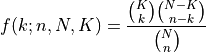# aesara.tensor.random.hypergeometric#

aesara.tensor.random.hypergeometric = <aesara.tensor.random.basic.HyperGeometricRV object>[source]#

A hypergeometric discrete random variable.

The probability mass function for hypergeometric for the number of successes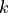in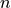draws without replacement, from a finite population of size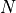with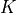desired items is: